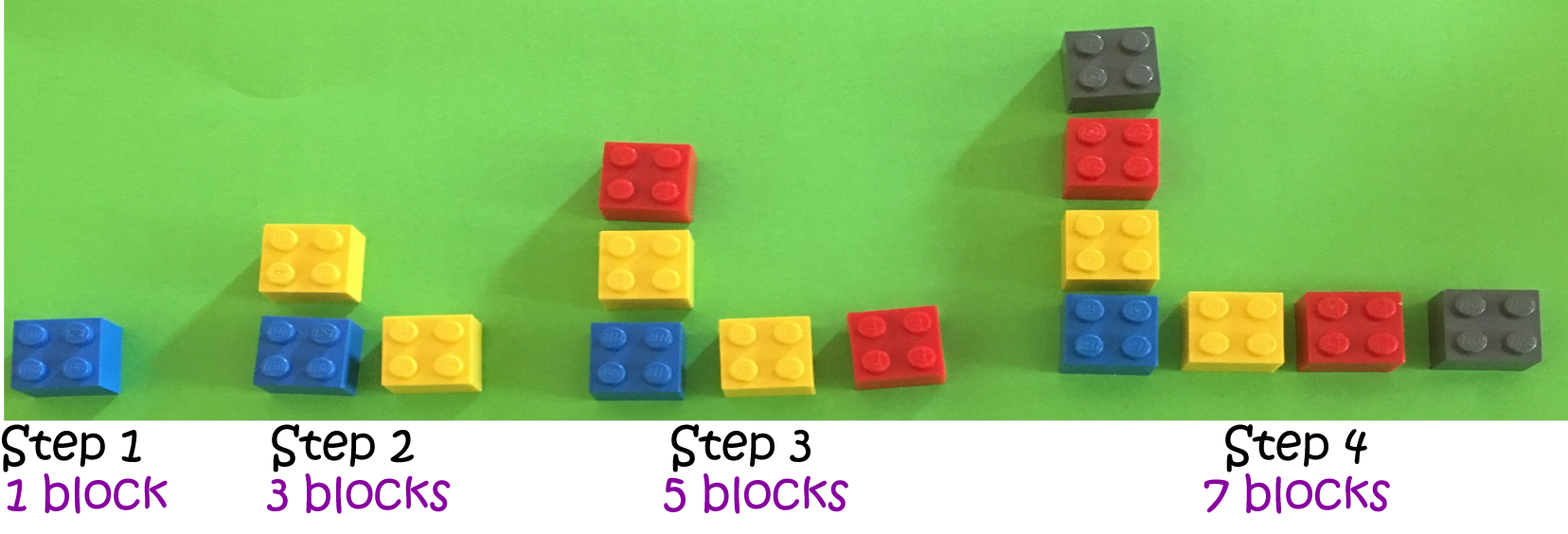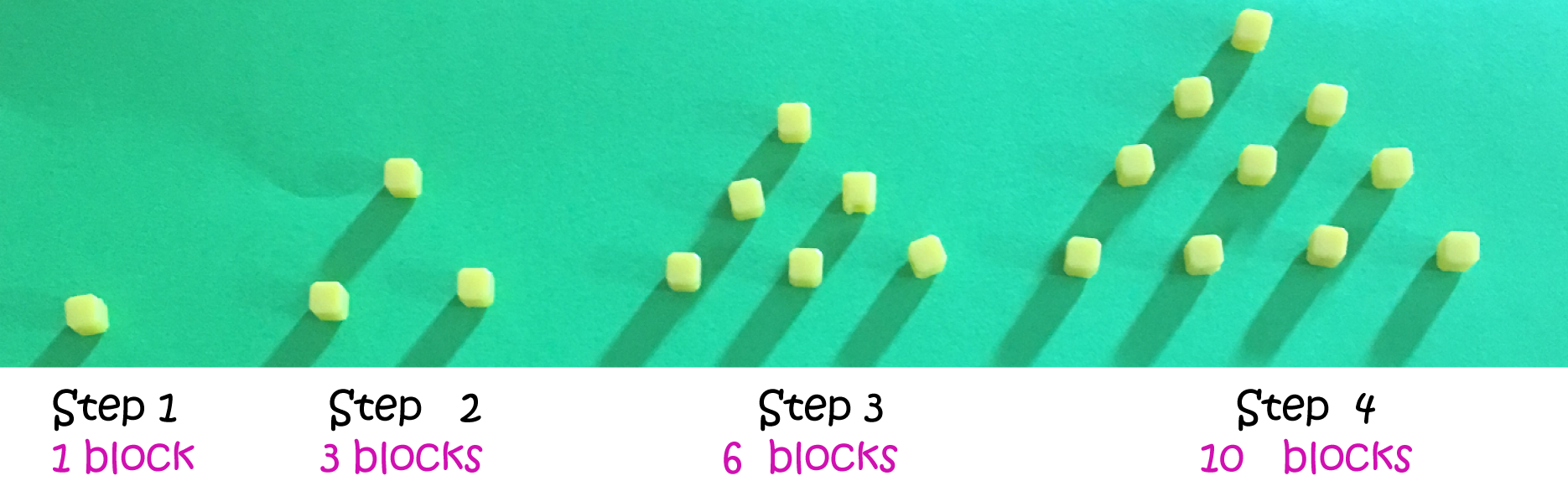# Building Your Own Number Patterns?

You can use just about anything you find around the house or garden to build your own number patterns: blocks, coins, seashells, gumnuts, toothpicks - whatever you like. You could even use the peas that are served up for dinner, but your parents mightn't be too happy to see you playing with your food.

Here's an example of a pattern built out of blocks:How many blocks are needed for step 5?

Can you come up with a formula that will tell you how many blocks are needed for each step? Notice that two blocks are added to each step to form the next step. So, the formula might look something like this:

$\text{Number of Blocks} = \text{(Some number)} \times \text{(Step Number)} + \text{(another number)} - \text{(a third number)}$.

I came up with this formula:
$\text{Number of Blocks} = 2\times \text{(Step Number)} + 1 - 2$.

Let's test it out for the steps that are shown:
 Step Number Number of Blocks Number given by formula 1 1 $2 \times 1 + 1 - 2 = 1$ 2 3 $2 \times 2 + 1 - 2 = 3$ 3 5 $2 \times 3 + 1 - 2 = 5$ 4 7 $2 \times 4 + 1 - 2 = 7$
This is looking pretty good!

## Another Example

How about this pattern?Notice that step 2 is obtained from step one by adding 2 blocks to it, step 3 is obtained from step 2 by adding three blocks to it, and step 4 is obtained from step 3 by adding 4 blocks to it. The numbers corresponding to the steps of this pattern are called the triangular numbers because you can arrange them to form triangles. It's not quite so easy to come up with a formula for this pattern, but why not have a try?

## A Third Example

The numbers in this pattern also have a special name:They're called the square numbers because they can be arranged to form squares, just like in the picture above. There's an easy formula for the numbers in this pattern. It's
$\text{Number of Blocks} = \text{(Step number)} \times \text{(Step Number)}$.

## Conclusion

Number patterns are found all over the place. Why not have a play and see if you can come up with some interesting patterns of your own? Just don't tell mum and dad that I was the one who said it was OK to play with your peas! That can be our little secret.

### Description

This mini book covers the core of Math for Foundation, Grade 1 and Grade 2 mathematics including

1. Numbers
3. Subtraction
4. Division
5. Algebra
6. Geometry
7. Data
8. Estimation
9. Probability/Chance
10. Measurement
11. Time
12. Money
13. and much more

This material is provided free of cost for Parent looking for some tricks for their Prekinder, Kinder, Prep, Year 1 and Year 2 children

### Learning Objectives

These lessons are for kids aged 4-8 with the core objective to expose their brains to concepts of addition, subtraction, division, algebra and much more.

Author: Subject Coach
You must be logged in as Student to ask a Question.